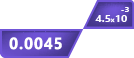# Standard Form Calculator

• Standard Version: 2000.02 x 10^2
• UK Version: 2000.02

Click To Copy

### Real Number :

--- Click To Copy

### Scientific Notation :

--- Click To Copy

### E-Notation :

--- Click To Copy

### Engineering Notation :

--- Click To Copy

## Standard form calculator

The standard form converter converts the given number to:

• Scientific notation
• Real number
• E-Notation, and
• Engineering notation

A standard notation converter accepts values both in exponential and decimal form and converts the input to standard notation in a heartbeat.

Keep on reading if you are interested in the standard form definition, how to find standard form manually, and a few examples of standard or scientific notation.

## How does this standard notation calculator work?

To convert numbers into the standard form follow the steps below:

• Enter the decimal or exponent number in the input box.
• Click the "Convert" button.
• The standard form calculator will convert the number into four different notations.
• Click the "reset" button if you want to recalculate.

After pressing the convert button this standard form calculator will convert your decimal or exponent number into the standard form and also gives the result in real numbers, scientific notation, E notation, and Engineering notation.

## What is the standard form?

Standard Form is a term in mathematics that is used for converting long or complex numbers into the simplest form for reducing the difficulty to read the number.

It is a way to express very small or very long numbers.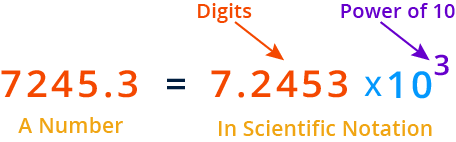The scientific notation calculator can be used for converting complex numbers into a readable format.

A large mathematical number like the given below is difficult to read. But, after it is converted into the standard form, it becomes easier to read.

Number: 5630000000000

Standard Form: 5.63 x 1012

Here is an example of the conversion of small numbers into the standard form:

Number: 0.0000067833

Standard Form: 6.7833-6

## How to write in the standard form manually?

Below, we have explained the step-by-step conversion of a number into the standard format.

Example:

Convert 0.000381 to standard form.

Solution:

Step 1: Write down the number.

0.000381

Step 2: Find the decimal point in the number and if the decimal point is not there then it would be declared at the end of the number, on the right side.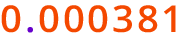Step 3: Place the decimal after the first non-zero digit and remove any leading or trailing zeros. In this case: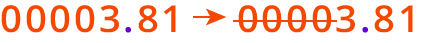The number becomes,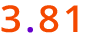Step 4: Now, count the number of positions you have changed for the decimal. Raise the total number of positions moved, to the power of 10. In this case, we have moved the decimal to 4 positions.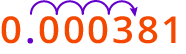Keynote: If the decimal is moved from left to right, place the negative sign with the exponent. If the decimal is moved from right to left, raise the power as a positive integer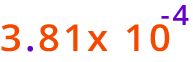So,

0.000381 = 3.81 × 10-4

Use the standard notation calculator to cross-check the answers.

FAQS

## What is the standard form of a Million?

The standard form of 1 million is 1 x 106.

## What is Standard Fraction?

The Standard Form of the fractions is known as the Standard Fraction. When the numerator and denominator are co-prime then it is considered to be written in the standard form. But for this, both the numbers shouldn’t have a common factor of 1 For example. 5/6, 11/12.

## What is the standard form of the quadratic function?

The general way of writing the quadratic function is:

f(x) = ax2 + bx + c

In Standard form, it is written as:

f(x) = a(x-h)2 + k